Search by Topic

Filter by: Content type:
Age range:
Challenge level:

There are 21 NRICH Mathematical resources connected to Maximise/minimise/optimise, you may find related items under Functions and Graphs.

Broad Topics > Functions and Graphs > Maximise/minimise/optimise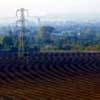Quick Route

Age 16 to 18 Challenge Level:

What is the quickest route across a ploughed field when your speed around the edge is greater?Exponential Trend

Age 16 to 18 Challenge Level:

Find all the turning points of y=x^{1/x} for x>0 and decide whether each is a maximum or minimum. Give a sketch of the graph.Discrete Trends

Age 16 to 18 Challenge Level:

Find the maximum value of n to the power 1/n and prove that it is a maximum.Where to Land

Age 14 to 16 Challenge Level:

Chris is enjoying a swim but needs to get back for lunch. If she can swim at 3 m/s and run at 7m/sec, how far along the bank should she land in order to get back as quickly as possible?Largest Product

Age 11 to 14 Challenge Level:

Which set of numbers that add to 10 have the largest product?A Close Match

Age 16 to 18 Challenge Level:

Can you massage the parameters of these curves to make them match as closely as possible?Catalyse That!

Age 16 to 18 Challenge Level:

Can you work out how to produce the right amount of chemical in a temperature-dependent reaction?Cyclic Triangles

Age 16 to 18 Challenge Level:

Make and prove a conjecture about the cyclic quadrilateral inscribed in a circle of radius r that has the maximum perimeter and the maximum area.Slippage

Age 14 to 16 Challenge Level:

A ladder 3m long rests against a wall with one end a short distance from its base. Between the wall and the base of a ladder is a garden storage box 1m tall and 1m high. What is the maximum distance. . . .Biggest Enclosure

Age 14 to 16 Challenge Level:

Three fences of different lengths form three sides of an enclosure. What arrangement maximises the area?Corridors

Age 14 to 16 Challenge Level:

A 10x10x10 cube is made from 27 2x2 cubes with corridors between them. Find the shortest route from one corner to the opposite corner.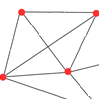Only Connect

Age 11 to 14 Challenge Level:

The graph represents a salesman’s area of activity with the shops that the salesman must visit each day. What route around the shops has the minimum total distance?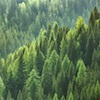Tree Tops

Age 14 to 16 Challenge Level:

Can you make sense of information about trees in order to maximise the profits of a forestry company?Little and Large

Age 16 to 18 Challenge Level:

A point moves around inside a rectangle. What are the least and the greatest values of the sum of the squares of the distances from the vertices?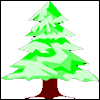Christmas Trees

Age 11 to 14 Challenge Level:

Christmas trees are planted in a rectangular array. Which is the taller tree, A or B?Find the Fake

Age 14 to 16 Challenge Level:

There are 12 identical looking coins, one of which is a fake. The counterfeit coin is of a different weight to the rest. What is the minimum number of weighings needed to locate the fake coin?Three Ways

Age 16 to 18 Challenge Level:

If x + y = -1 find the largest value of xy by coordinate geometry, by calculus and by algebra.Without Calculus

Age 16 to 18 Challenge Level:

Given that u>0 and v>0 find the smallest possible value of 1/u + 1/v given that u + v = 5 by different methods.Quartics

Age 16 to 18 Challenge Level:

Investigate the graphs of y = [1 + (x - t)^2][1 + (x + t^)2] as the parameter t varies.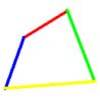Biggest Bendy

Age 16 to 18 Challenge Level:

Four rods are hinged at their ends to form a quadrilateral. How can you maximise its area?Real(ly) Numbers

Age 16 to 18 Challenge Level:

If x, y and z are real numbers such that: x + y + z = 5 and xy + yz + zx = 3. What is the largest value that any of the numbers can have?